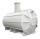# Vintner

How high can vintner fill keg with crushed red grapes if these grapes occupy a volume of 20 percent? The keg is cylindrical with a diameter of the base 1 m and a volume of 9.42 hl. Start from the premise that says that fermentation will fill the keg (the number П equals 3.14).

h =  0.2399 m

### Step-by-step explanation:Did you find an error or inaccuracy? Feel free to write us. Thank you!Tips to related online calculators
Do you want to convert area units?
Do you know the volume and unit volume, and want to convert volume units?

#### You need to know the following knowledge to solve this word math problem:

We encourage you to watch this tutorial video on this math problem:

## Related math problems and questions:

• ContainerThe container has a cylindrical shape the base diameter 0.8 m and the area of the base is equal to the area of the wall. How many liters of water can we pour into the container?
• Height as diameter of baseThe rotary cylinder has a height equal to the base diameter and a surface of 471 cm2. Calculate the volume of a cylinder.
• Cylindrical tankCylindrical tank holds 600hl water and is deep 2.5 m. Calculate the diameter of the cylinder.
• Cylindrical tank9.6 hl of water is poured into a cylindrical tank with a bottom diameter of 1.2 m. What height in centimeters does the water reach?
• Volume and surfaceCalculate the volume and surface area of the cylinder when the cylinder height and base diameter is in a ratio of 3:4 and the area of the cylinder jacket is 24 dm2.
• Cylinder containerThe cylindrical container with a diameter of 1.8 m contains 2,000 liters of water. How high does the water reach?
• Equilateral cylinderEquilateral cylinder (height = base diameter; h = 2r) has a volume of V = 199 cm3 . Calculate the surface area of the cylinder.
• Cylinder:Cylinder: calculate the height of a 3 liter cylindrical pot with a diameter of 10 cm.
• Perimeter of baseThe circumference of the base of the rotating cylinder is same as its height. What is the diameter and height of this cylinder with volume 1 liter?
• The cylinder baseThe cylinder with a base of 8 dm2 has a volume of 120 liters. From a cylinder filled with water, 40 liters of water was removed. At what height from the bottom /with precision to dm/ is the water level?
• Iglu - cone tentThe cone-shaped tent is 3 m high, the diameter of its base is 3.2 m. a) The tent is made of two layers of material. How many m2 of fabric is needed for production (including flooring) if 20% needs to be added to the minimum amount due to cutting waste? b)
• The potThe pot is a cylinder with a volume of V = 7l and an inner diameter of d = 20cm. Find its depth.
• Fuel economyHow many kilometers is sufficient petrol in the cylinder fuel tank with a diameter of 40 cm and the base of tank length of 1 m, when it is filled to 60%, and if the car consumes 15 liters per 100 km?
• CylinderIn a 1-meter diameter cylinder is 1413 liters of water, which is 60% of the cylinder. Calculate the cylinder height in meters, do not write the units. The resulting value round and write as an integer.
• Tank diameterA cylindrical tank has a volume of 60 hectoliters and is 2.5 meters deep. Calculate the tank diameter.
• Cylinder heightCalculate the height of the cylinder and its surface is 2500 dm2 and the bases have a diameter 5dm.
• Ratio of volumesIf the heights of two cylindrical drums are in the ratio 7:8 and their base radii are in the ratio 4:3. What is the ratio of their volumes?